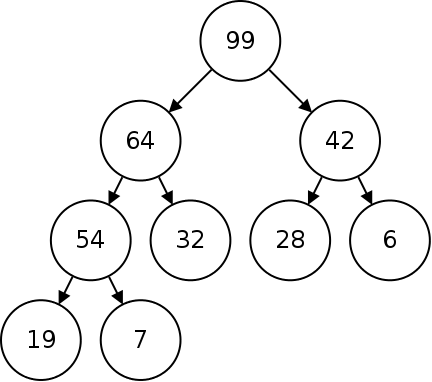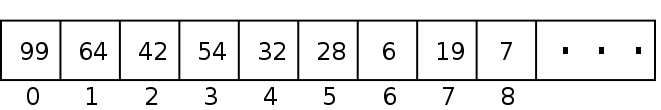# Heaps

### Priority Queues

A queue is a data structure for storing elements in a first-in-first-out manner. So we will remove items in the same order that they came in.

In some applications, however, we may want to allow elements to skip others in some situations, such as task scheduling.

Another use of priority queues is when we want to choose from among a set of objects based on some metric. For example, in Prim's algorithm, we choose edges with the smallest weight, and in Dijkstra's we choose nodes with the least current tentative cost.

In a priority queue, we assign each object a priority, and when it comes time to take an object from the queue, we take the one with the highest priority. If there are multiple at the same priority level, we will take the oldest (using FIFO).

We could use an array or linked list to implement a priority queue. To insert an object into the queue, we will add it to the end. Then to remove one, we will scan through searching for the oldest one with the highest priority level.

A more efficient implementation is based on a heap.

### Heaps

A heap is a type of binary tree where each node's value is greater than or equal to the value of each of its children. Heaps are also perfectly balanced with the nodes in the last level in the leftmost positions. The following is an example of a heap:This heap is called a max heap because the root node is the maximum. We could also have a min heap where each node's value is less than or equal to that of its children.

Here we will only talk about max heaps, though the same ideas apply to min heaps as well.

Using a heap to represent a priority queue, we will always take the root item next as this is the one with the largest priority.

Notice that there is no particular relationship between sibling nodes, the only relationship that matters is the parent to the child.

### Operations

Heaps support a number of different operations:

• Creation - Create an empty heap.
• Insert - Add an element to the heap.
• Next - Return the root element.
• Remove - Remove the root element from the heap.

### Implementing a Tree with an Array

How these are implemented depends on how the heap is implemented. We can implement trees using a linked structure as we did for binary search trees.

However, we can also implement a tree with an array. Doing this with a heap, we will store the root in array location 0, then the two children of the root in the next two locations. The rule that the heap must be balanced and left-aligned implies that there is only one array location where each item can be stored.With a linked tree, we can find the left and right children by following the node pointers in each node. In an array we need to calculate the location of each child. Notice that the levels in the tree are stored consecutively with each level having twice the size:To find the left child of a node, what formula can we use?





What formula can we use for the right child?





What about the parent node?





### Insertion

To insert an item into the heap, we will need to maintain the heap property. To do this we will start by inserting the item into the next available array location, and then fix the heap. Fixing the heap after an insertion is called upheaping.The above heap has an element (in red) inserted in the next available location. To fix the heap, we will test if the item we inserted is less than its parent. If not we will swap the node with its parent.In the above heap, we swapped 83 with its parent 32. We will repeat this process until the parent is greater, or the node we inserted becomes the root.This heap shows the final result of inserting 83.

The algorithm for insertion is given below:

1. Insert the item at the next available location.
2. While the item is not the root, and it has a greater value than its parent:
1. Swap the item with its parent.

Below is a method for insertion for a Heap class:


// insert an item into the heap
void insert(type item) {
// put the new one in the next slot
array[count] = item;
int node = count;
int p = parent(node);
count++;

// upheap it until it is under a node which is bigger than it (or the root)
while ((node != 0) && (array[node] > array[p])) {
type temp = array[node];
array[node] = array[p];
array[p] = temp;

node = p;
p = parent(node);
}
}


What is the Big-O of the insertion algorithm?





### Removing the Top

When we want to find the max element, it will be stored in the top of the heap. Typically, when we look at the top of the heap, we will also want to remove that item. In the heap below, for example, we would want to remove the root element, 99.After deletion, we need to maintain the property that the heap is fully left aligned. This means that the spot we need to remove is the rightmost one in the bottom level.So to delete the root, we will swap it with the last element in the bottom level (as illustrated above). Then we will remove the last element in the bottom. Finally we will fix the heap by downheaping.

To downheap, we will check if the item is bigger than both children, if not we will swap it with its larger child and repeat. This is illustrated below:In pseudocode:
1. Save the root as the return value.
2. Move the last child to the root.
3. While one of the children of this node has a greater value:
1. Find the largest of the children.
2. Swap the largest child with this node.
4. Return the original value.

In C++:


// remove the top item
type dequeue() {
// save the item to return it
type value = array;

// move the last item to the root and decrement
array = array[count - 1];
count--;

// find the children
int node = 0;
int l = left(node);
int r = right(node);

// while one of the children is bigger
while(((l < count) && (array[l] > array[node])) ||
((r < count) && (array[r] > array[node]))) {

// find the larger node
int max;

// if there are two, take larger
if((l < count) && (r < count)) {
if(array[l] > array[r])
max = l;
else
max = r;
}
// if just one take that one
else if(l < count) {
max = l;
} else {
max = r;
}

// swap them
type temp = array[max];
array[max] = array[node];
array[node] = temp;

// update the indices
node = max;
l = left(node);
r = right(node);
}

return value;
}


What is the Big-O of the removal algorithm?





### Priority Queues

Heaps provide a very efficient way of implementing priority queues.

Using an array or linked list, enqueueing is $O(1)$, but we would have to search through for the highest priority item using $O(N)$ time to dequeue.

Alternatively we could use a sorted array or linked list to achieve $O(1)$ dequeue, but to insert and maintain the order would require $O(N)$ for enqueue.

As discussed, inserting into a heap is $O(\log N)$ and removing the top node is also $O(\log N)$.

To implement a priority queue, we can associate some priority with each data item. The heap will use these priority values to maintain the heap.

This example implements a priority queue in this way. This program stores numbers in the priority queue and prioritizes them in different levels. The highest priority level is prime, then even, then odd. The program uses 3, 2, and 1 for this purpose.

### Heap Sort

Heaps also provide a way of sorting data. We can add the data into a heap and then take it back out again (which will be in order). This algorithm is given below:

1. For each data item:
1. Add it to a heap.
2. While the heap isn't empty:
1. Use the top as the next item.
2. Remove the top.

What is the Big-O of Heap Sort?